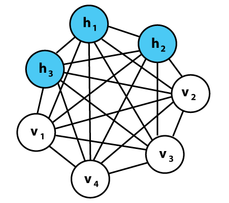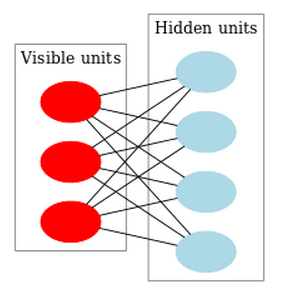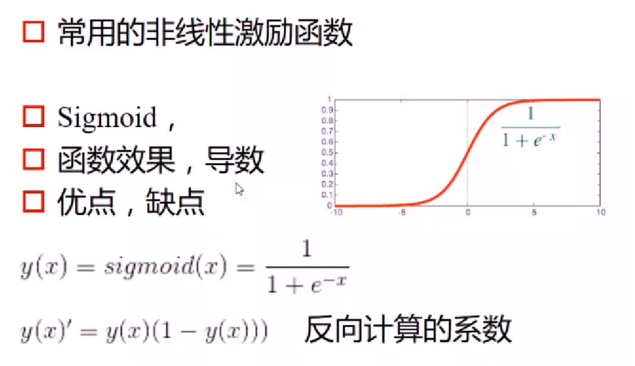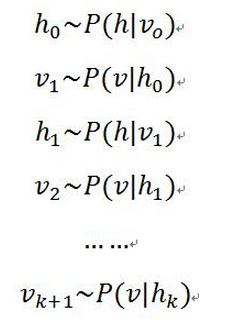# 受限玻尔兹曼机(Restricted Boltzmann Machine)分析

## 1、什么是BM？

BM是由Hinton和Sejnowski提出的一种随机递归神经网络，可以看做是一种随机生成的Hopfield网络，是能够通过学习数据的固有内在表示解决困难学习问题的最早的人工神经网络之一，因样本分布遵循玻尔兹曼分布而命名为BM。BM由二值神经元构成，每个神经元只取1或0这两种状态，状态1代表该神经元处于接通状态，状态0代表该神经元处于断开状态。在下面的讨论中单元和节点的意思相同，均表示神经元。1、递归神经网络（RNN）本质是学习一个映射关系，因此有输入和输出层的概念，而玻尔兹曼机的用处在于学习一组数据的“内在表示”，因此其没有输出层的概念。
2、递归神经网络各节点链接为有向环，而玻尔兹曼机各节点连接成无向完全图。

## 2、什么是RBM？RBM中，所有可见单元和隐单元之间存在连接，而隐单元两两之间和可见单元两两之间不存在连接，也就是层间全连接，层内无连接（这也是和玻尔兹曼机BM模型的区别，BM是层间、层内全连接）。其中，每一个节点（无论是Hidden Unit还是Visible Unit）都有两种状态：处于激活状态时值为1，未被激活状态值为0。

RBM本质是非监督学习(Unsupervised Learning)的利器（Hinton和吴恩达都认为：将来的机器学习任务慢慢都会转变为非监督学习的），因为，它可以用于降维（隐层少一点），学习特征（隐层输出就是特征），自编码器（AutoEncoder）以及深度信念网络（多个RBM堆叠而成）等。

## 3、RBM

Wij$W_{ij}$： 表示第i个可见单元和第j个隐单元之间的权值

ai$a_i$： 表示第i个可见单元的偏置阈值

bj$b_j$： 表示第j个隐单元的偏置阈值

E(v,h|θ)=i=1naivij=1mbjhji=1nj=1mviWijhj

P(v,h|θ)=eE(v,h|θ)Z(θ)

Z(θ)=v,heE(v,h|θ)

P(v|θ)=1Z(θ)heE(v,h|θ)

1）在给定可见单元的状态时，各隐藏层单元的激活状态之间是条件独立的。此时，第j个隐单元的激活概率为：

P(hj=1|v)=f(bj+iviWij)

2）相应的，当给定隐单元的状态时，可见单元的激活概率同样是条件独立的：

P(vi=1|h)=f(ai+jWijhj)

f$f$为激励函数/激活函数，这里选用sigmoid作为激活函数。是因为它可以把(-∞，+∞)的值映射到[0,1]这个区间。也就是说，无论模型的可见层输入节点数据处于一个多大的范围内，都可以通过sigmoid函数求得它相应的函数值，即节点的激活概率值。## 4、RBM模型参数求解

P(v|θ)=1Z(θ)heE(v,h|θ)$P(v|\theta) = \frac {1} {Z(\theta)} \sum_{h}e^{-E(v,h|\theta)}$

• 首先是似然函数的对数形式ln(P(vt))$ln(P(v^{t}))$vt$v^{t}$表示模型的输入数据。

• 然后对参数Wij,ai,bj$W_{ij}, a_i, b_j$分别进行求导，以Wij$W_{ij}$为例：

ln(P(vt))wij=P(hj=1|vt)vtivP(v)P(hj=1|v)vi

ln(P(vt))ai=vtivP(v)vi

ln(P(vt))bj=P(hj=1|vt)vP(v)P(hj=1|v)

## 5、Gibbs采样算法

Gibbs采样的思想是：虽然不知道一个样本数据x=(x1,x2,...,xn)$x = (x_1, x_2, ... , x_n)$的联合概率P(x)$P(x)$，但是知道样本中每一个数据的条件概率P(xi|x1,x2,...,xi1,xi+1,...,xn)$P(x_i|x_1,x_2,...,x_{i-1},x_{i+1},...,x_n)$ （假设每一个变量都服从一种概率分布），则我可以先求出每一个数据的条件概率值，得到x的任一状态 [x1(0),x2(0),...,xn(0)]$[x_1(0), x_2(0), ..., x_n(0)]$。然后，我用条件概率公式迭代对每一个数据求条件概率。最终，迭代k次的时候，x的某一状态[x1(k),x2(k),...,xn(k)]$[x_1(k), x_2(k), ..., x_n(k)]$ 将收敛于x的联合概率分布P(x)$P(x)$Gibbs采样过程

P(hj=1|v0)=f(bj+iv0viWij)
h0$h_0$中每个节点的条件概率；

P(vi=1|h0)=f(ai+jh0Wijhj)

v1$v_1$中每个节点的条件概率，然后依次迭代，直到执行K步（K足够大），此时P(v|h)$P(v|h)$的概率将收敛于P(v)$P(v)$的概率。如下所示：## 参考文献